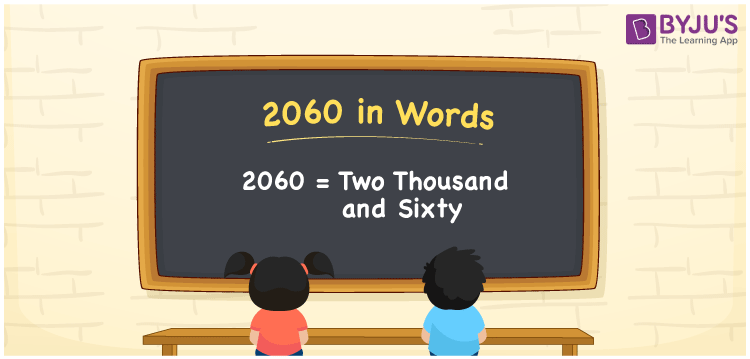# 2060 in Words

2060 in words can be written as Two Thousand and Sixty. Students will be able to learn the conversion of 2060 in words which will help them understand the applications of numbers in our daily lives. If you buy a mixer for Rs. 2060, then you can say that “I have bought a mixer for Two Thousand and Sixty Rupees”. The  number 2060 can be written in words using the English alphabet. The numbers in words can be grasped easily by the students using the resources given at BYJU’S. 2060 in English can be read as “Two Thousand and Sixty”.

 2060 in words Two Thousand and Sixty Two Thousand and Sixty in Numbers 2060

## 2060 in English Words## How to Write 2060 in Words?

Students will learn about the conversion of 2060 into words from place value charts. The number 2060 has four digits. For 2060, the place value chart is prepared in a table form to help students understand it effectively.

 Thousands Hundreds Tens Ones 2 0 6 0

2060 in expanded form is explained in brief here:

2 × Thousand + 0 × Hundred + 6 × Ten + 0 × One

= 2 × 1000  + 0 × 100 + 6 × 10 + 0 × 1

= 2000 + 60

= 2060

= Two Thousand and Sixty

Therefore, 2060 in words is written as Two Thousand and Sixty.

2060 is a natural number that precedes 2061 and succeeds 2059.

2060 in words – Two Thousand and Sixty

Is 2060 an odd number? – No

Is 2060 an even number? – Yes

Is 2060 a perfect square number? – No

Is 2060 a perfect cube number? – No

Is 2060 a prime number? – No

Is 2060 a composite number? – Yes

## Frequently Asked Questions on 2060 in Words

Q1

### How do you write 2060 in words?

2060 can be written as “Two Thousand and Sixty” in words.
Q2

### Is 2060 an even number?

2060 is an even number because it is divisible by 2.
2060/2 = 1030
Q3

### How can Two Thousand and Sixty be written in numbers?

Two Thousand and Sixty can be written in numbers as 2060.